## 基于碰撞模型的斜坡滚石颗粒速度预测 1)

*兰州理工大学土木工程学院, 兰州 730050

## VELOCITY PREDICTION OF SLOPE ROLLING STONE PARTICLE BASED ON COLLISION MODEL 1)

Wei Xinrong*,, Duan Shaozhen,*,,2), Sun Jinlong*,, Wang Wenda*,

*School of Civil Engineering,Lanzhou University of Technology,Lanzhou 730050,China

The Key Laboratory of Disaster Prevention and Mitigation in Civil Engineering of Gansu Province,Lanzhou University of Technology,Lanzhou 730050,China

 基金资助: 1)国家自然科学基金.  11702124

Received: 2020-02-15   Accepted: 2020-04-29   Online: 2020-05-18Abstract

The landslide rolling stone disaster is a prevailing natural hazard in the western mountainous regions of China. The rolling stone disasters featured by burstiness and uncertainty are hard to forecast and prevent the geological disaster. Based on the particle contact theory and considering the random factors in the process of the particle collision, a theoretical model for predicting the velocity of the rolling stone particles after impacting the slope is established. According to the theorem of impulse and momentum moment, the basic equations of rolling stone particles collision are established, and the analytical solutions of the rebound velocity after the rolling stone particles impacting the slope are obtained. The results show that the analytical solutions of the rebound velocity after impacting include the random factors such as slope angle, the velocity and angle of particles on the slope, incident velocity, angle and impact angle. It is found that the model results agree well with the experimental results when the impact angle of incident rolling stone particle impacts the particle on the slope is changed. At the same time, this paper predicts the probability distribution of the rebound velocity, rebound angle and rebound rotational angular velocity after the collision. The results show that the probability distributions of rebound velocity, rebound angle and rebound rotation angular velocity follow Gaussian distribution. When the velocity of particles on the slope and the slope angle change, they have no qualitative influence on the rebound particle velocity, rebound angle and the rebound rotation angular velocity probability distribution, but they have significant influence on the center parameters of probability distribution.

Keywords： rolling stone ; particle collision ; contact model ; slope ; probability distributions

Wei Xinrong, Duan Shaozhen, Sun Jinlong, Wang Wenda. VELOCITY PREDICTION OF SLOPE ROLLING STONE PARTICLE BASED ON COLLISION MODEL 1). Chinese Journal of Theoretical and Applied Mechanics[J], 2020, 52(3): 707-715 DOI:10.6052/0459-1879-20-039

## 1 滚石颗粒随机撞击模型的建立

### 图1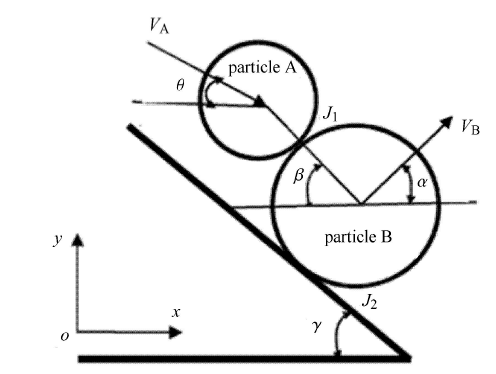Fig.1   The model of rock impacting on the slope

### 1.2 基本方程的建立

(1) 对于滚石A和B的碰撞压缩阶段,即：从碰撞开始到碰撞压缩量达到最大时,所遵循的力学方程如下：

$S'_\tau = m_{A} [U'_{{A}x} \sin \beta + U_{{A}y} \cos \beta - V_{A} \sin (\beta -\theta )]$

$S'_n = m_{A} [U'_{{A}y} \sin \beta - U'_{{A}x} \cos \beta + V_{A} \cos (\beta -\theta )]$

$S'_\tau R_{A} = \dfrac 12 m_{A} \omega' _{A} R_{A}^2$

$S'_1 \cos \gamma + S'_2 \sin \gamma + S'_n \cos \beta - S'_\tau \sin \beta = \\ \qquad m_{B} (U'_{{B}x} - V_{B} \cos \alpha )$

$S'_2 \cos \gamma - S'_1 \sin \gamma - S'_n \sin \beta - S'_\tau \cos \beta = \\ \qquad m_{B} (U'_{{B}y} - V_{B} \sin \alpha )$

$(S'_1 + S'_\tau )R_{B} = \dfrac{1}{2}m_{B}\omega' _{B} R_{B}^2$

(2) 颗粒A和B碰撞完成后整个碰撞过程所遵循的力学方程如下：

$S_\tau = m_{A} [U_{{A}x} \sin \beta + U_{{A}y} \cos \beta - V_{A} \sin (\beta - \theta )]$

$S_n = m_{A} [U_{{A}y} \sin \beta - U_{{A}x} \cos \beta + V_{A} \cos (\beta -\theta )]$

$S_\tau R_{A} = \dfrac 12 m_{A} \omega _{A} R_{A}^2$

$S_1 \cos \gamma + S_2 \sin \gamma + S_n \cos \beta - S_\tau \sin \beta = \\ \qquad m_{B} (U_{{B}x} - V_{B} \cos \alpha )$

$S_2 \cos \gamma - S_1 \sin \gamma - S_n \sin \beta - S_\tau \cos \beta = \\ \qquad m_{B} (U_{{B}y} - V_{B} \sin \alpha )$

$(S_1 + S_\tau )R_{B} = \dfrac{1}{2}m_{B} \omega _{B} R_{B}^2$

### 1.3 连接条件

$x$方向的平衡方程

$U'_{{A}x} \cos \beta - U'_{ {A}y} \sin \beta = U'_{{B}x} \cos \beta - U'_{{B}y} \sin \beta$

$y$方向的平衡方程

$\omega'_{{A}} R_{A} + U'_{{A}x} \sin \beta + U'_{{A}y} \cos \beta = \\ \qquad - \omega'_{B} R_{B} + U'_{{B}x} \sin \beta + U'_{{B}y} \cos \beta$

$x$方向的平衡方程

$U'_{{B}x} \sin \gamma + U'_{{B}y} \cos \gamma = 0$

$y$方向的平衡方程

$\omega'_{B} R_{B} + U'_{{B}x} \cos \gamma - U'_{{B}y} \sin \gamma = 0$

$S_n = (1 + k_1 )S'_n$
$S_\tau = (1 + k_2 )S'_\tau$
$S_1 = (1 + k_2 )S'_1$
$S_2 = (1 + k_1 )S'_2$

## 2 结果分析

### 2.1 滚石-壁面碰撞模型验证与分析

Table 1  Comparison of test data and model calculation data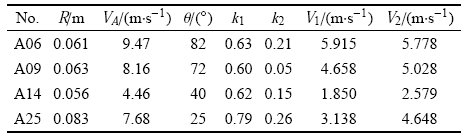Notes: VA is the experimental incident velocity, V1 is the experimental rebound velocity, and V2 is the theoretical rebound velocity.

### 图2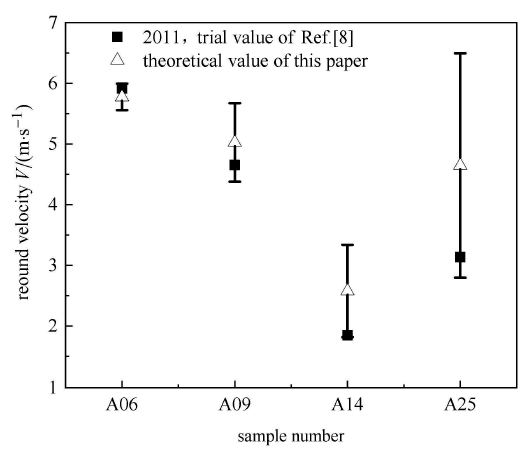Fig.2   Comparison of theoretical results with experimental results

### 图3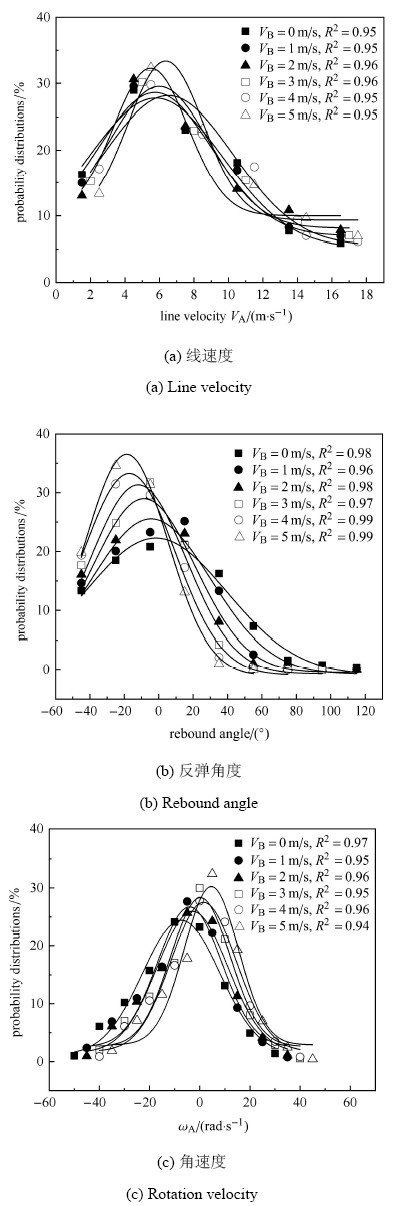Fig.3   Rebound particle velocity and angular probability distribution after collision when the particle B' velocity is different ($V_{B} =0$,1,2,3,4,5 m/s, $R_{A}=R_{B}=0.2$ m)

### 图4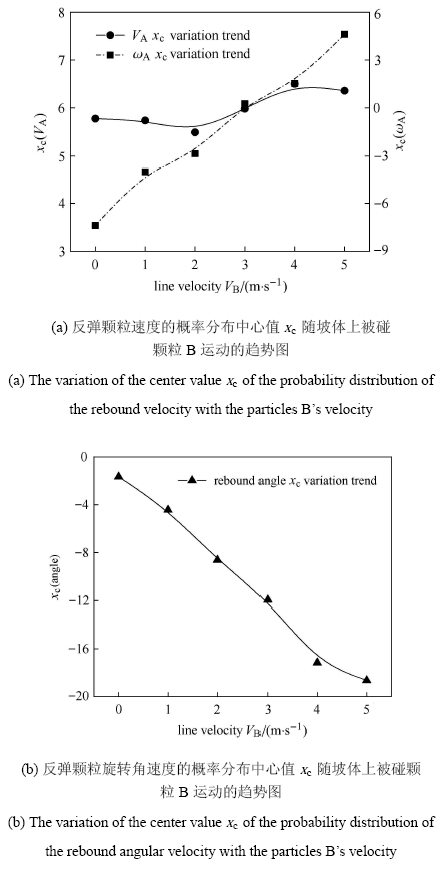Fig.4   The variation of the center value $x_{c}$ of the probability distribution of the rebound velocity and angular velocity with the particles B velocity ($V_{B}=0$,1,2,3,4,5 m/s, $R_{A}=R_{B} =0.2$ m)

### 图5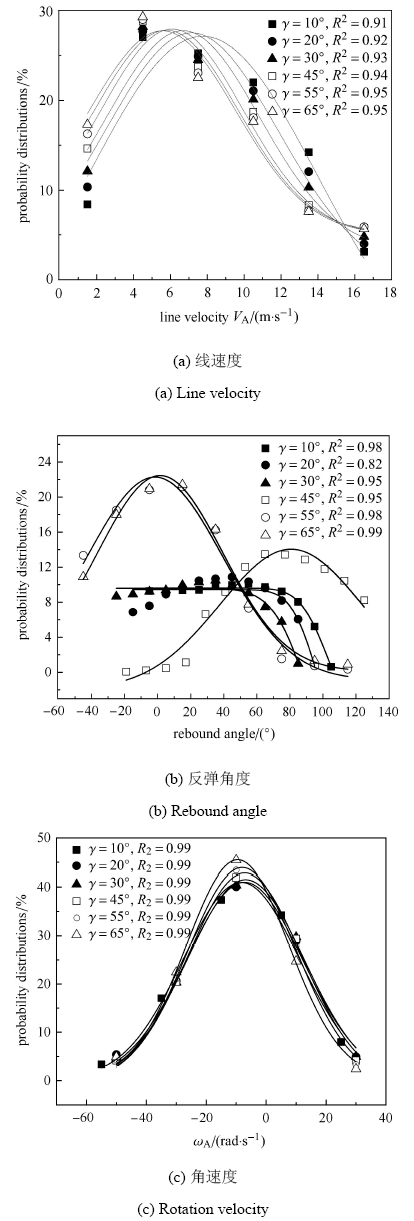Fig. 5   Probability distribution of rebound particle velocity and rotational angular velocity on a stationary slope with different slope angles ($\gamma =10^\circ$,20$^\circ$,30$^\circ$,45$^\circ$,55$^\circ$,65$^\circ$,$R_{A}=R_{B} =0.2$ m)

### 图6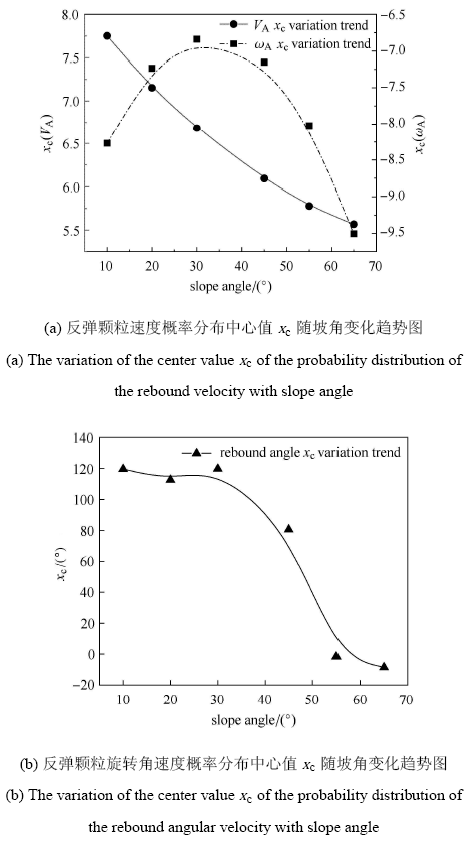Fig.6   The variation of the center value $x_{c}$ of the probability distribution of the rebound velocity and angular velocity with slope angle ($\gamma =10^\circ$,20$^\circ$,30$^\circ$,45$^\circ$,55$^\circ$,65$^\circ$,$R_{A}=R_{B} =0.2$ m)

## 3 结论与讨论

### 3.1 结论

(1) 本文建立了普适的斜坡滚石碰撞模型,该碰撞模型可描述滚石与斜坡、滚石与滚石、滚石与平板,滚石与运动床面的碰撞过程.

(2) 得到了滚石碰撞后反弹滚石颗粒速度解析解,这一解析解包含了影响滚石碰撞过程的6个随机因素,即：坡角、坡体上被碰颗粒B的速度和角 度、入射速度和角度、撞击角度等随机因素.

(3) 该理论模型结果显示,反弹滚石速度和角度以及反弹旋转角速度的概率分布均服从高斯分布形式,坡体上被碰滚石颗粒B的速度和坡角对反弹滚石速度和角度以及反弹旋转角速度的概率分布定性上没有影响,但对高斯分布的中心值参数有显著影响.

### 3.2 讨论

/

 〈〉